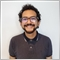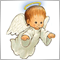# Help with my EA.932

I just ended up a MQL4 course and started to mess around with Meta Editor, just a simple MA crossover EA, but I'm having a few problems:

1- I want the EA to open a trade on the next crossover, not on the current one.

2- I can't get the EA to close the first order and then open another one.

The course was damn basic, I think the issue is related to the void Something() stuff, because it was not seen on the code we made, we only use OnTick function, and the system was oriented to work only on a certain hour, so I think I'm trying to code a different kind of system using the wrong logic.

Here's the coding:

```extern int MASlow   = 30;
extern int MAFast   = 25;
extern int MASignal = 10;
extern double Lots  = 0.01;
extern double StopLoss = 30; // StopLoss (0 = Cerrar en señal contraria).
extern double TakeProfit = 30; // TakeProfit (0 = Cerrar en señal contraria).
enum Digitos_Broker
{
Cuatro, //Cuatro Digitos
Cinco  //Cinco Digitos
};
input Digitos_Broker Digitos = Cinco; // Digitos en el brokker.

//+------------------------------------------------------------------+
//| Expert initialization function                                   |
//+------------------------------------------------------------------+
int OnInit()
{
return(INIT_SUCCEEDED);
}

//+------------------------------------------------------------------+
//| Expert tick function                                             |
//+------------------------------------------------------------------+
void OnTick()
{
static double SlowMA = iMA(Symbol(),Period(),MASlow,0,MODE_LWMA,PRICE_CLOSE,1);
static double FastMA = iMA(Symbol(),Period(),MAFast,0,MODE_LWMA,PRICE_CLOSE,1);
static double SignalMA = iMA(Symbol(),Period(),MASignal,0,MODE_LWMA,PRICE_CLOSE,1);
static int ticket = 0;
//====Conversion de 4 a 5 digitos====//
double Pips = Point;
if(Digitos == Cinco)
{
Pips = Point*10;
}
else if(Digitos == Cuatro)
{
Pips = Point;
}
//====Lotaje o Cerrar en entrada opuesta====//
double TradeLots = Lots;
if(Lots > 0)
{
TradeLots = Lots;
}
else if(Lots == 0)
{
TradeLots = 0;
}
//=====Revisar trades=====//
bool res;
res = OrderSelect(ticket,SELECT_BY_TICKET);
//====Enviar trades====//
if(res == false)
{
if (SignalMA > FastMA && SignalMA > SlowMA)
{
ticket = OrderSend(Symbol(),OP_BUY,TradeLots,Ask,50,0,0,"RKSDTV");

if(ticket < 0)
{
Alert("Error enviando orden de compra");
Alert("Codigo del error: ",GetLastError());
}
}
else if (SignalMA < FastMA && SignalMA < SlowMA)
{
ticket = OrderSend(Symbol(),OP_SELL,TradeLots,Bid,50,0,0,"RKSDTV");
if(ticket < 0 )
{
Alert("Error enviando orden de venta");
Alert("Codigo del error: ",GetLastError());
}
}
}
else if(res == true)
{
if(OrderCloseTime() == 0)
{
if(OrderType() == OP_BUY)
{
if(SignalMA < FastMA && SignalMA < SlowMA)
{
bool res3;
res3 = OrderClose(ticket,TradeLots,OrderClosePrice(),50);
if (res3 == false)
{
Alert("Error cerrando orden #",ticket);
Alert("Codigo del error: ",GetLastError());
}
}
}
else if(OrderType() == OP_SELL)
{
if(SignalMA > FastMA && SignalMA > SlowMA)
{
bool res4;
res4 = OrderClose(ticket,TradeLots,OrderClosePrice(),50);
if(res4 == false)
{
Alert("Error cerrando orden #",ticket);
Alert("Codigo del error: ",GetLastError());
}
}
}
}
}
}```4659

FernandoBorea:

1- I want the EA to open a trade on the next crossover, not on the current one.

What do you mean by the next crossover?4659

FernandoBorea:

2- I can't get the EA to close the first order and then open another one.

You can't close the order by using OrderClosePrice() (returned value is zero, because the order is still open). You have to use Ask or Bid.

EDIT: I'm sorry, I was wrong. You can use OrderClosePrice() and it is a better way.4659

FernandoBorea:

Here's the coding:

Why do you use this? The variable "Pips" isn't use in your code! You can delete the whole block.

```//====Conversion de 4 a 5 digitos====//
double Pips = Point;
if(Digitos == Cinco)
{
Pips = Point*10;
}
else if(Digitos == Cuatro)
{
Pips = Point;
}
```

Similar problem. Result of this block is TradeLots is equal to Lots. You should use just Lots. You can delete the whole block.

```//====Lotaje o Cerrar en entrada opuesta====//
double TradeLots = Lots;
if(Lots > 0)
{
TradeLots = Lots;
}
else if(Lots == 0)
{
TradeLots = 0;
}
```

You shouldn't use this coding

```if(res == false)
...
else if(res == true)
```

You should use this instead of

```if(!res)
...
else
...```932

Petr Nosek:

What do you mean by the next crossover?

Sorry, I'm not too good at english, for example, let's say signal MA is over Slow and Fast one, when I attach the EA it immediately opens the trade even though the crossover was some bars ago.932

Petr Nosek:

You can't close the order by using OrderClosePrice() (returned value is zero, because the order is still open). You have to use Ask or Bid.

Can you explain me that?4659

FernandoBorea:
Sorry, I'm not too good at english, for example, let's say signal MA is over Slow and Fast one, when I attach the EA it immediately opens the trade even though the crossover was some bars ago.

Because you don't check crossover but just the position of SignalMA. If you want to check crossover you should compare the previous position with the current position.932

Petr Nosek:

Why do you use this? The variable "Pips" isn't use in your code! You can delete the whole block.

Similar problem. Result of this block is TradeLots is equal to Lots. You should use just Lots. You can delete the whole block.

You shouldn't use this coding

You should use this instead of

Pips variable it's for 4 to 5 broker digits conversion.

Lots was a mistake I just noticed, I posted an old version, that block was just to test on some logical conditions when I was on the course. I was going to use the structure to set SLLevel and TPLevel because if I set it to 0 on the variable then because of the Bid +/- SL/TP*Pips would place the SL at entry

Could you please explain me the difference between using (!res) and then else? What does " ! " Do?4659

FernandoBorea:
Can you explain me that?

OrderClosePrice() returns close price. If you close the order for example at 1.23000 the OrderClosePrice() will return 1.23000. If the order is still open the OrderClosePrice() returns 0.0 . Similar to OrderCloseTime()

EDIT: see the post #2932

Petr Nosek:

I've edited your code but not tested:

What's the Magic Number for?4659

FernandoBorea:
Pips variable it's for 4 to 5 broker digits conversion.

OK, but you never use "Pips" in your code behind the block.